# Definition of Polygon

Definition of Polygon
Go back to  'Shapes'

Polygons have a wide variety of shapes and are classified based on the number of sides they have.

The most common ones are the triangle, the square, and the rectangle.## Lesson Plan

 1 What is the Definition of Polygon? 2 Important Notes on Polygons 3 Solved Examples on Polygons 4 Thinking Out of the Box! 5 Interactive Questions on Polygons

## What is the Definition of Polygon?

A polygon is a closed two-dimensional figure, that comprises three or more straight lines.

Common examples of polygons are triangles, squares, pentagons, hexagons, etc.

The table shown below illustrates some of the polygon examples.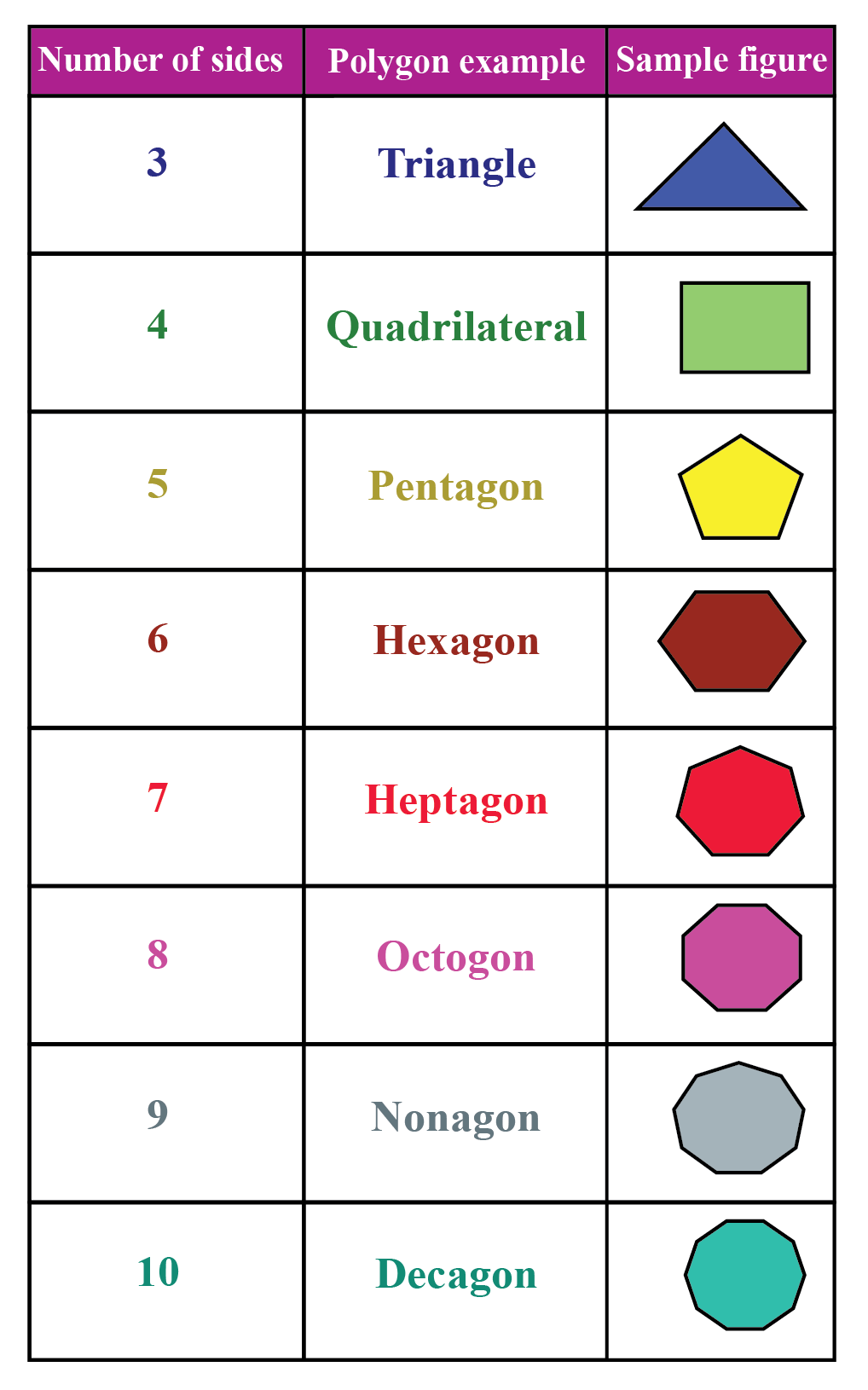## What Are the Different Types of Polygons?

There are six different types of polygons. They are:

• Regular polygons
• Irregular polygons
• Concave polygons
• Convex polygons
• Simple polygons
• Complex polygons

### Regular Polygons

Regular polygons are polygons whose length is equal on all sides and which include equilateral angles.

Examples: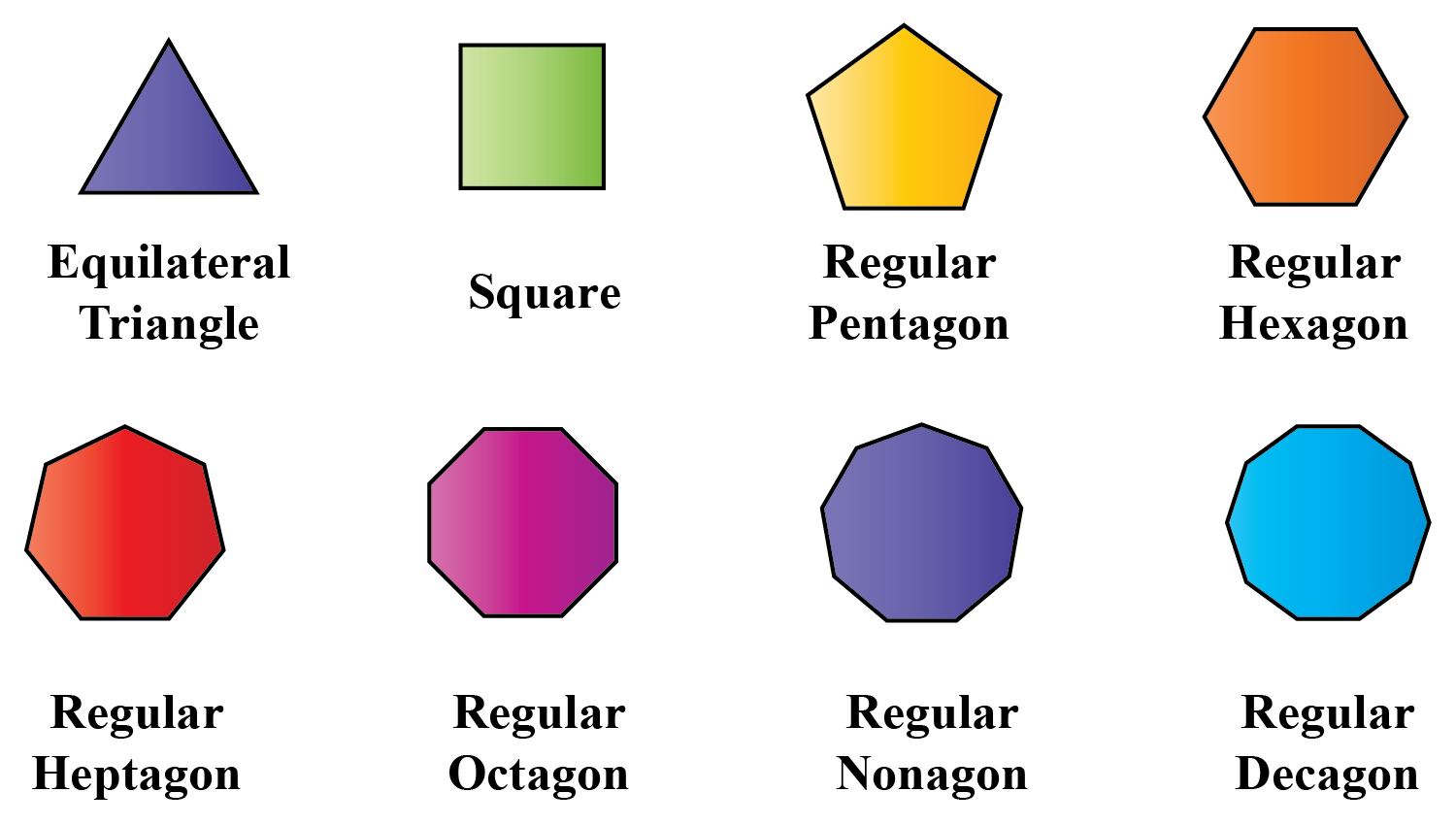### Irregular Polygons

These polygons do not have sides of equal length and the angles differ from each other.

Examples: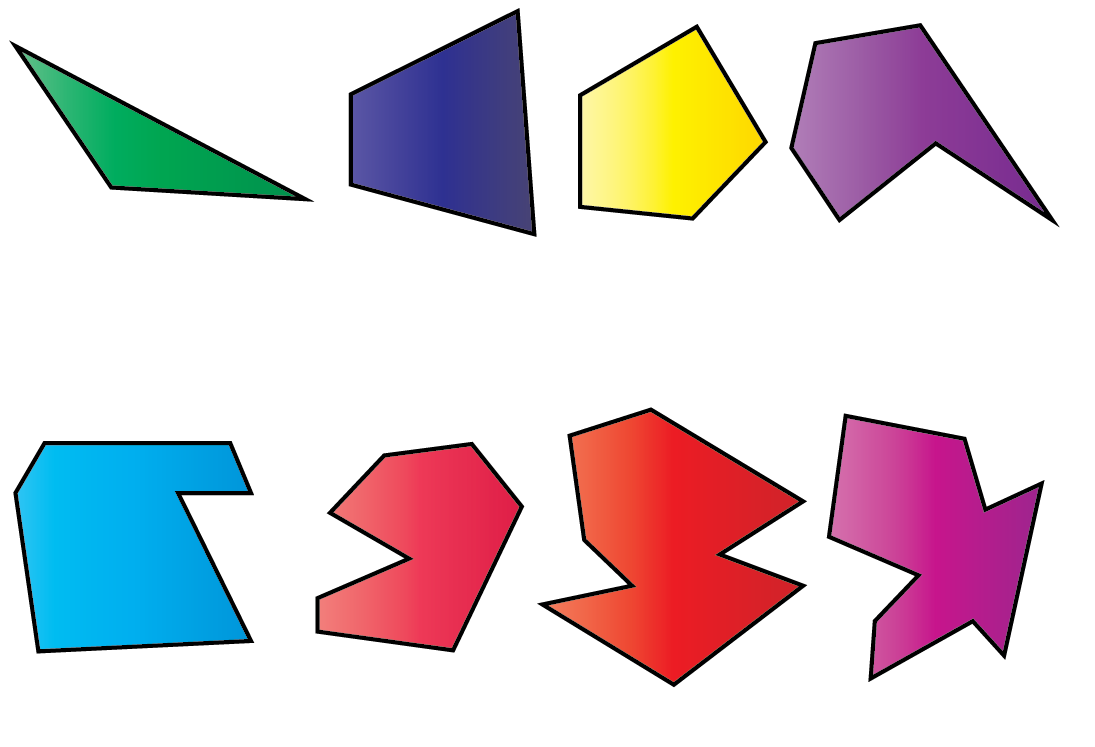Even though the number of sides is equal in both regular and irregular polygons, the length of the sides and the interior angles differ.

This table illustrates the differences between regular and irregular polygons.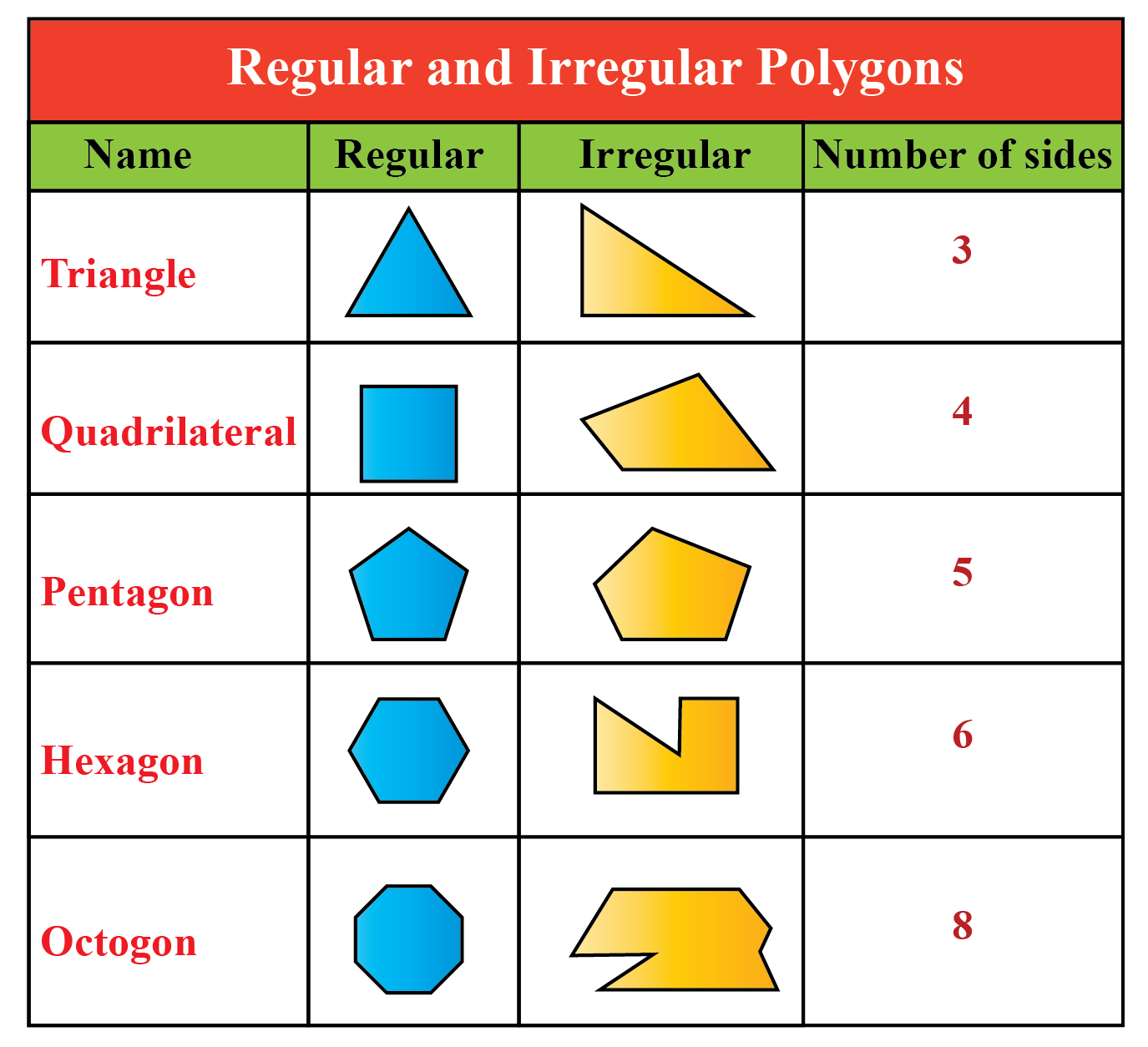### Concave Polygons

Polygons in which at least one of the interior angles is greater than 180º are called concave polygons.

Examples: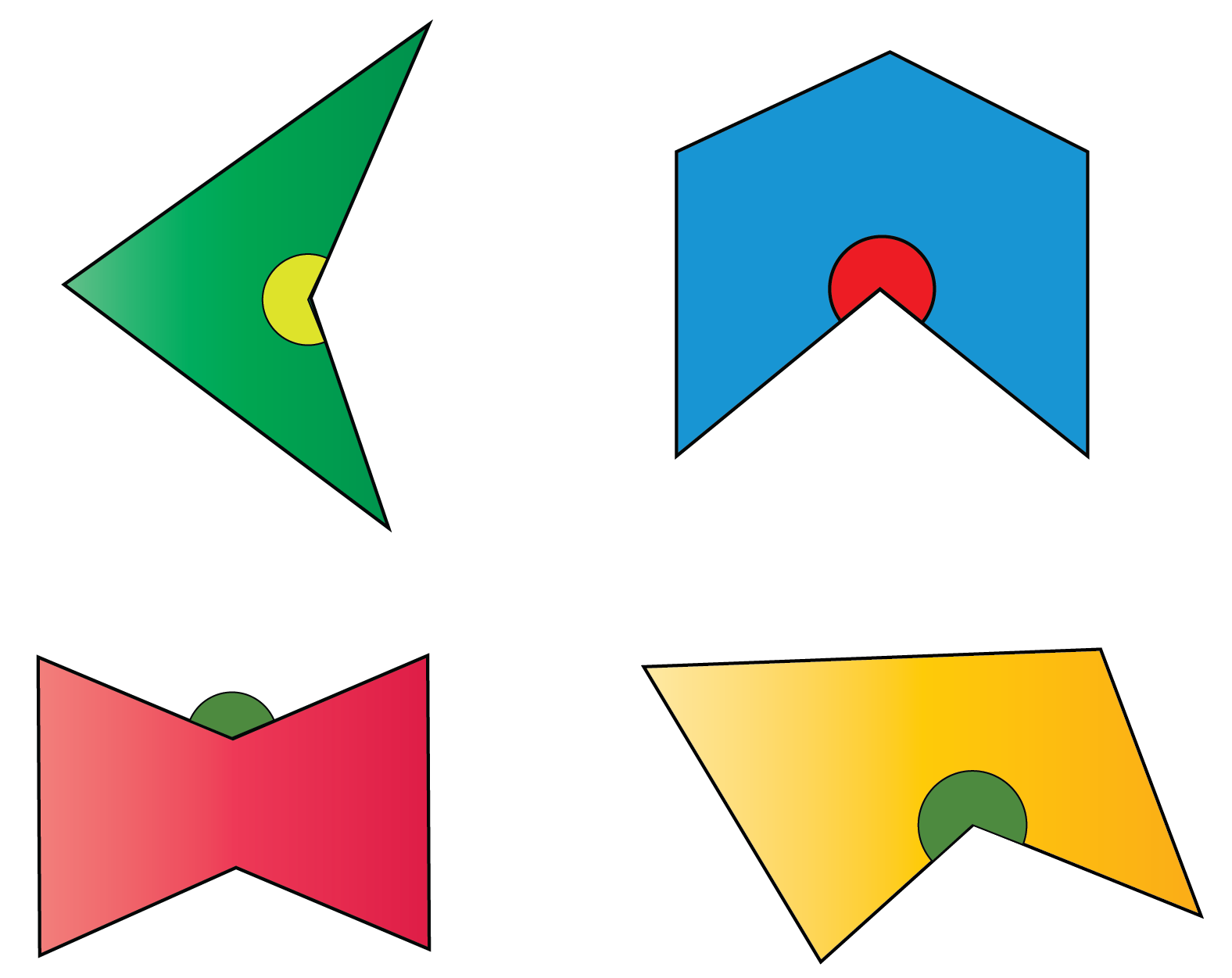The angle represented here denotes that one of the interior angles is greater than 180º.

### Convex Polygons

Polygons in which every interior angle is less than 180º are called convex polygons.

In other words, a polygon which is not concave is called a convex polygon.

Examples: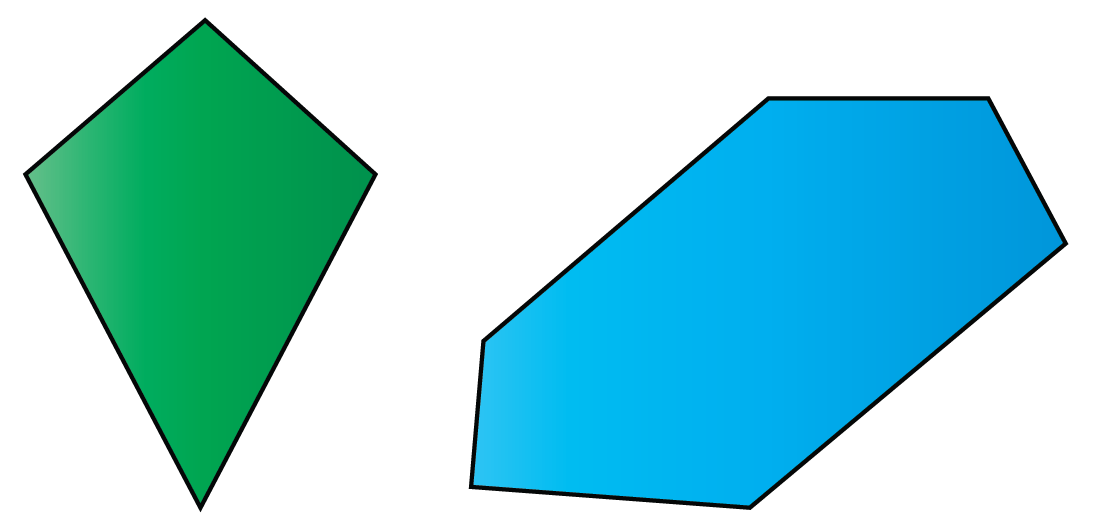Polygons are classified as concave or convex based on their interior angles.

Concave polygons will have at least one of their interior angles measuring greater than 180º, whereas convex polygons will have all their interior angles measuring less than 180º.

### Simple Polygons

A simple polygon is a polygon that does not intersect itself.

A simple polygon consists of one boundary.

Examples:### Complex Polygons

Polygons that intersect themselves and have more than one boundary are called complex polygons.

Examples: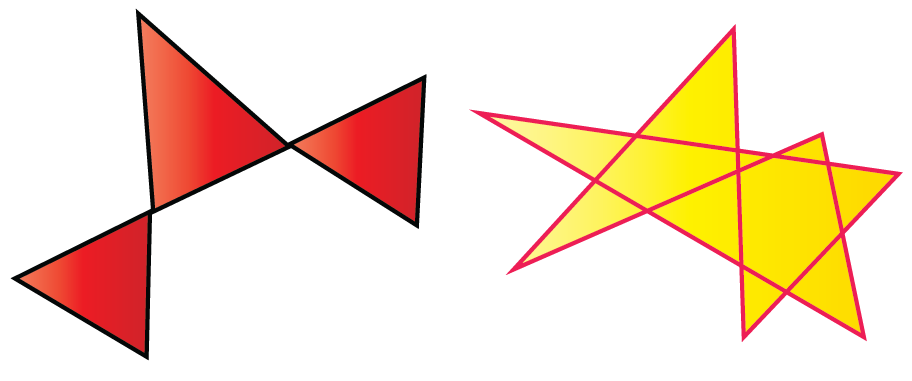## What Are Regular Polygons?

### Regular Polygon: Definition

Regular polygons are polygons whose length is equal on all sides and that have equal interior angles.

Regular polygons are convex i.e., all the interior angles measure less than 180º.

Some images of real-life examples of regular polygons are given below.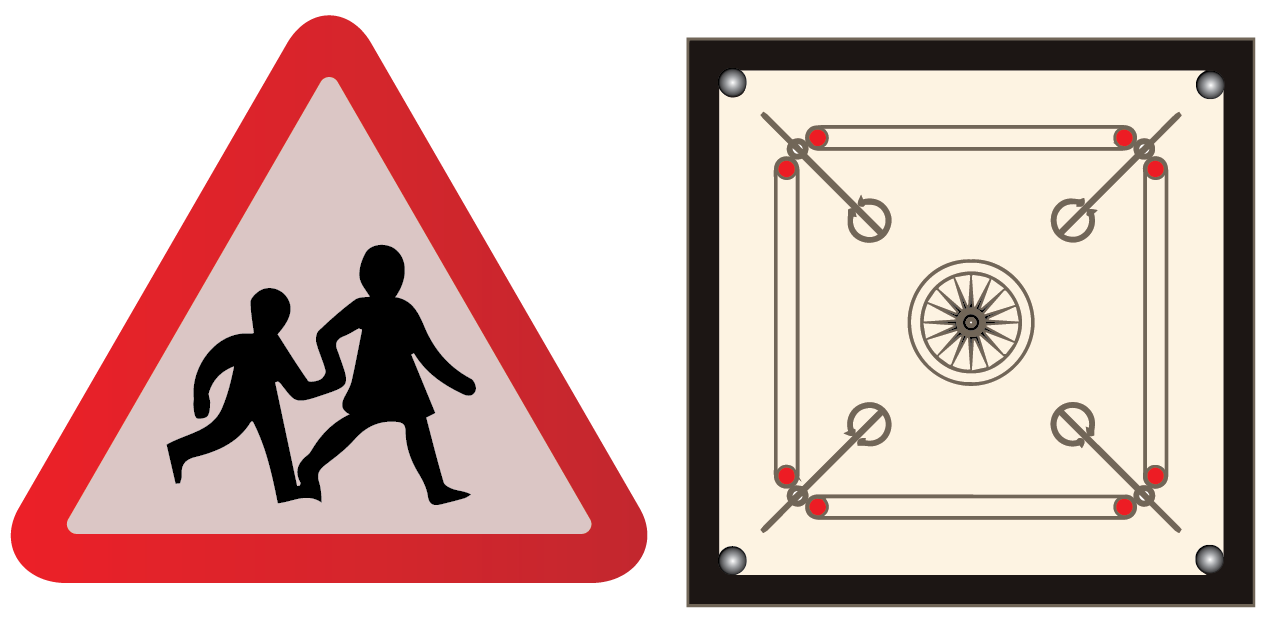Look around you and try to find some regular polygons.Challenging Questions

1. Is a concave polygon a regular polygon?

2. Is a circle a polygon?

## What Are the Angles of a Regular Polygon?

We have learnt that regular polygons have equal interior angles.

Now we will learn about two types of angles in regular polygons.

• Exterior angles
• Interior angles

### Exterior Angle

An exterior angle is an angle formed between any side and the line extended to its adjacent side.

In regular polygons, the sum of the exterior angles equals 360º i.e., it forms a circle outside.

The exterior angle at a vertex can be obtained by the following formula.

 \begin{align}\text{Exterior Angle} = \frac{360^{\circ}}{n}\end{align}

Where "n" denotes the number of sides.

Example:

What is the measure of an exterior angle of an equilateral triangle?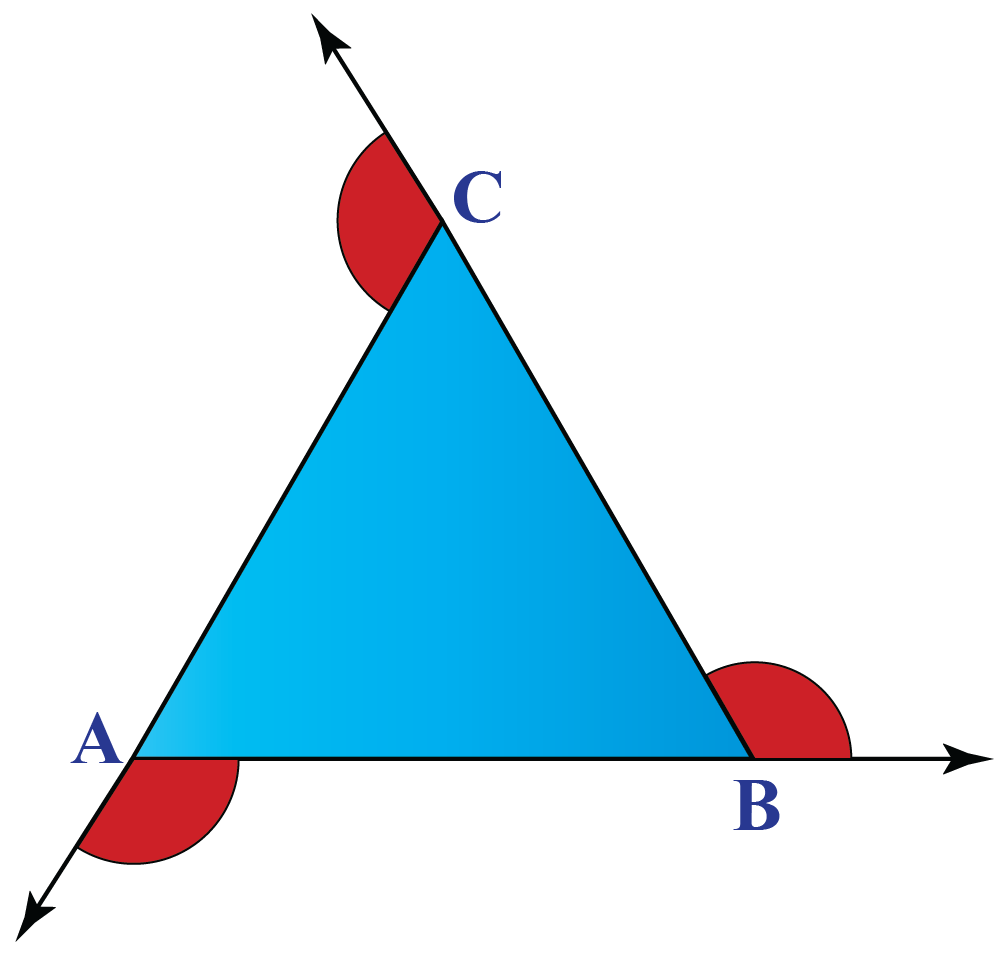Exterior angle at one vertex = $$\frac{360^{\circ}}{n}$$

A triangle has 3 sides i.e., n=3

Exterior angle = $$\frac{360^{\circ}}{3}$$

 $$\therefore$$ Exterior angle = 120º

The simulation shown below indicates the angle measure of the exterior angles of regular polygons.

Click on the names of the polygons to check the measure of each of their exterior angles.

### Interior Angle

An interior angle is an angle measured between the two sides of a polygon.

In regular polygons, the sum of the pair of exterior and interior angles equals 180º.

Therefore, the sum of the interior angle can be found by the following formula.

 $$\text{sum of the interior angles}=180^{\circ}(n-2)$$

The interior angle at a vertex can be found by the following formula.

 \begin{align}\text{Interior angle} = \frac{180^{\circ}(n-2)}{n}\end{align}

Example:

What is the interior angle of a regular pentagon?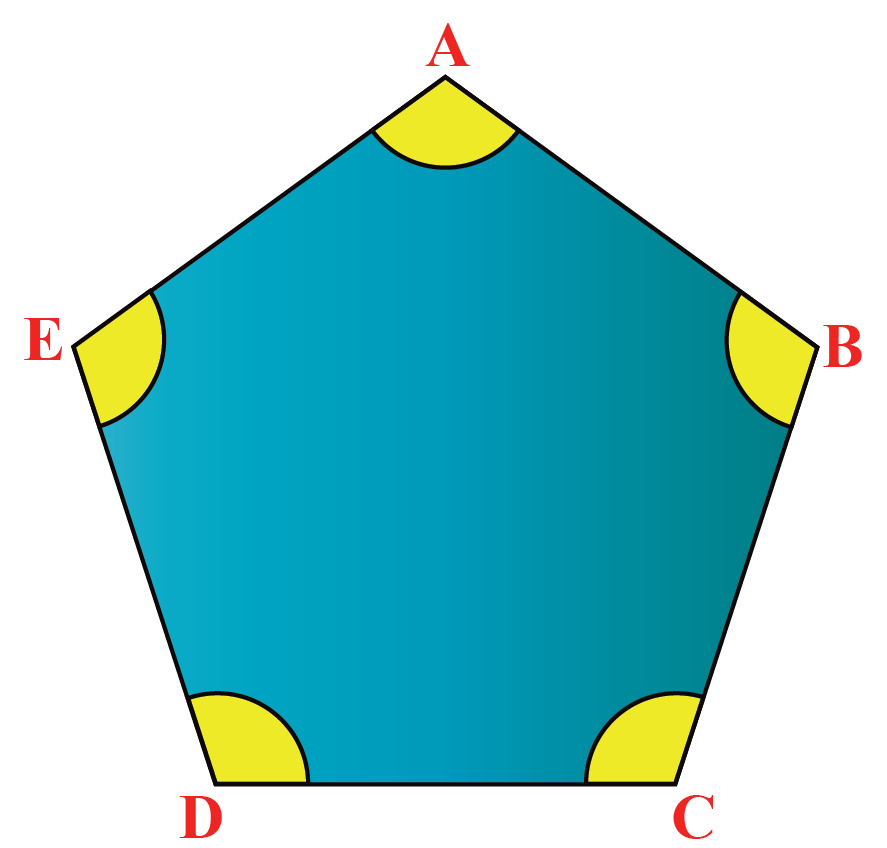Interior angle at one vertex =  $$\frac{180^{\circ}(n-2)}{n}$$

A pentagon has 5 sides i.e., n = 5

Interior angle = $$\frac{180^{\circ}(5-2)}{5}$$

 $$\therefore$$ Interior angle of a pentagon (one vertex) = 108º

This simulation will show you the angle measure of the interior angles of regular polygons.

Click on the names of the polygons to check the measure of each of their interior angles.

## Solved Examples

 Example 1

Help Rahul classify these polygons based on their shapes.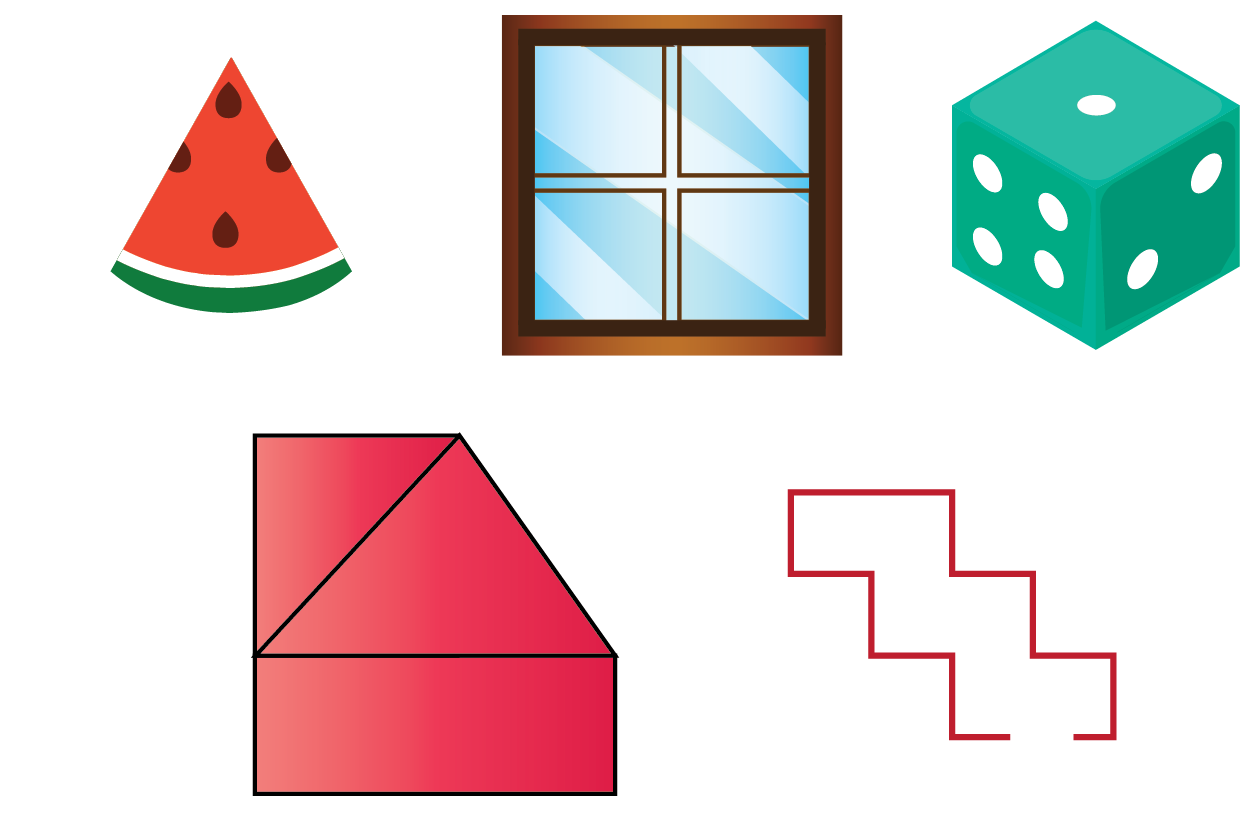Solution

The watermelon slice has one curved side. A polygon should have only straight lines. Thus, the slice of watermelon is not a polygon.

The dice is not a polygon because it is three dimensional. It is a polyhedron.

A polygon does not have any open ends. Therefore, the open-ended shape is not a polygon.

Hence, the polygons are: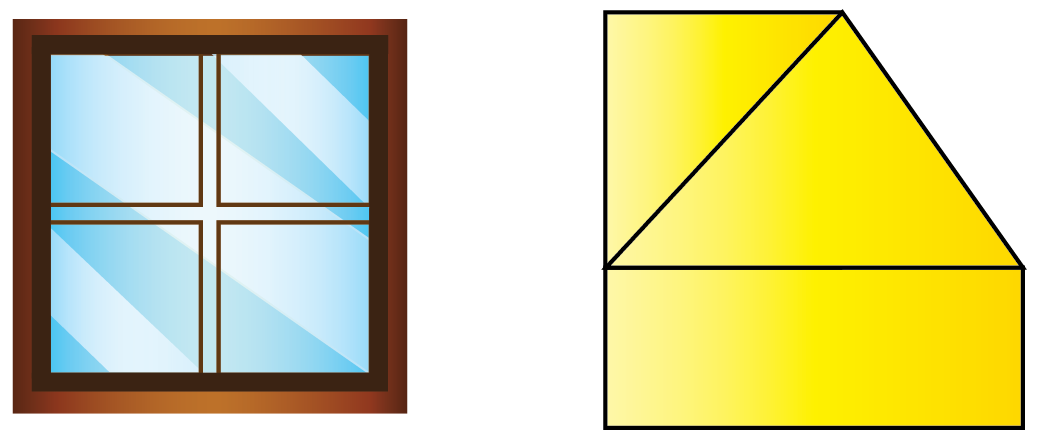$$\therefore$$ The window and the closed shape are polygons.
 Example 2

Anjali challenges Ria to identify the regular polygons from the shapes given below.

Can you help Ria win the challenge?SolutionAmong these four images, the photo frame has equal length on all sides and it includes equilateral angles.

Therefore, Ria must pick the photo frame.

 $$\therefore$$ The photo frame is a regular polygon.
 Example 3

Varun is doing his math homework.

The interior angle of a regular pentagon is given as 60º.

Help Varun find the exterior angle of the regular pentagon.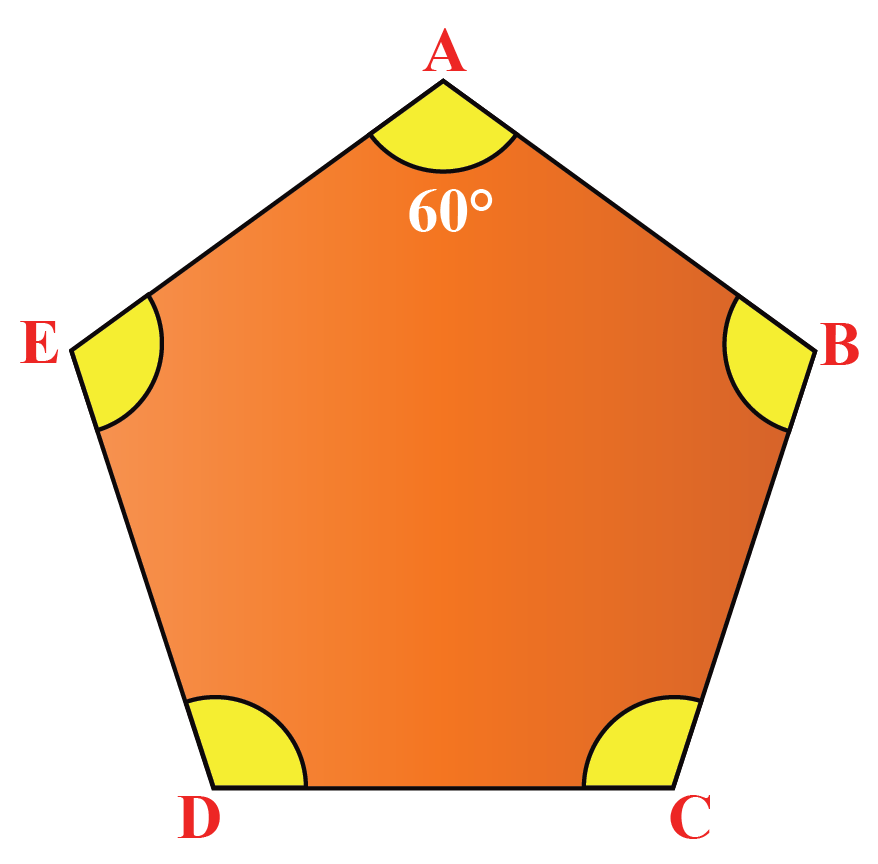Solution

We know that the sum of the interior angle and the exterior angle pair in a regular polygon equals 180º.

Therefore, $\text{Exterior angle} = 180^{\circ} - \text{interior angle}$

$\text{Exterior angle} = 180^{\circ} - 60^{\circ}$

 $$\therefore$$ Exterior angle of the regular pentagon = 120º
 Example 4

Aarav wants to find the exterior angle of a regular polygon that has 13 sides.

Help Aarav find the angle measure of the exterior angle.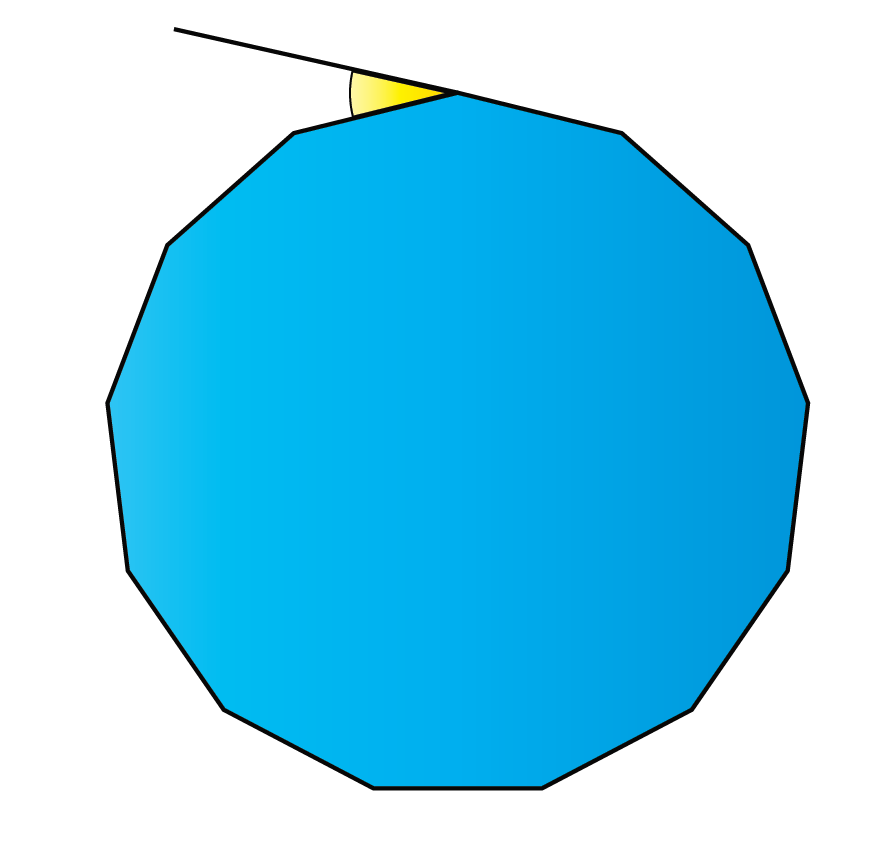Solution

The exterior angle of a regular polygon is,

$\text{Exterior Angle} = \frac{360^{\circ}}{n}$

where n = 13

$$\text{Exterior Angle} = \frac{360^{\circ}}{13}$$

 $$\therefore$$ Exterior angle of a 13-sided regular polygon = 27.69º.Think Tank

What is the name of the polygon that has 10,000 sides?

## Interactive Questions

Here are a few activities for you to practice.

## Let's Summarize

We hope you enjoyed learning about the definition of a polygon with the simulations and practice questions. Now you will be able to easily solve problems on the various types of polygon, polygon examples, sides, and recall the regular polygon definition.

At Cuemath, our team of math experts is dedicated to making learning fun for our favourite readers, the students!

Through an interactive and engaging learning-teaching-learning approach, the teachers explore all angles of a topic.

Be it worksheets, online classes, doubt sessions, or any other form of relation, it’s the logical thinking and smart learning approach that we at Cuemath believe in.

## 1. What is a polygon shape?

A polygon shape is a closed two-dimensional figure that consists of three or more definite straight lines.

## 2. What is the polygon formula?

The regular polygon formulae are:

\begin{align}\text{Exterior Angle} = \frac{360^{\circ}}{n}\end{align}

\begin{align}\text{Sum of the interior angles}=\\180^{\circ} -\frac{360^{\circ}}{n}\end{align}

\begin{align}\text{Interior angle} = \frac{180^{\circ}(n-2)}{n}\end{align}

## 3. Why are polygons called so?

The word "polygon" is derived from the terms "poly" which means "many" and "gon" which means "angle."

Therefore, a polygon denotes a shape with many sides and angles.

More Important Topics
Numbers
Algebra
Geometry
Measurement
Money
Data
Trigonometry
Calculus